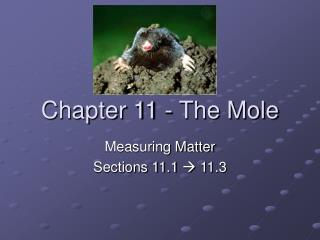DownloadDownload PresentationChapter 11 - The Mole

# Chapter 11 - The Mole

Download Presentation## Chapter 11 - The Mole

- - - - - - - - - - - - - - - - - - - - - - - - - - - E N D - - - - - - - - - - - - - - - - - - - - - - - - - - -
##### Presentation Transcript

1. Chapter 11 - The Mole Measuring Matter Sections 11.1  11.3

2. Measuring Matter One pair = 2One dozen = 12 One gross = 144One ream = 500

3. Measuring a Mole • Because atoms are so tiny, when chemists count atoms, they use a unit of measurement called a “mole” which equals 6.02 x 10 23 atoms. This is a very large number because it measures extremely tiny items. 602,000,000,000,000,000,000,000

4. Putting the Mole into Perspective • One mole of pennies would allow every person on Earth to spend a million dollars an hour, day and night, and still have half of it unspent when they died • One mole of popcorn would cover every dry surface of the Earth to a depth of six miles • One mole of stacked pennies would reach to the moon and back 16 times • One mole of marshmallows would cover the United States to a depth of 650 miles

5. History of Avogadro’s Number • Avogadro: 1811 – equal volumes of gases at the same temperature and pressure contain equal numbers of molecules • Cannizzaro: 1861 – used Avogadro’s hypothesis, developed a set of atomic weights for the known elements based on comparing the weights of equal volumes of gas • Loschmidt: 1865 – determined the number of atoms present in a given volume of air • Ostwald: 1902 – developed the concept of the mole • 1971 – the true value, 6.02x1023, was actually determined and is called Avogadro’s number since the entire string of events started with his findings in 1811

6. Molecular Mass of Atoms • Determined by number of protons and neutrons it contains • Example 1: Hydrogen atom – has one proton and therefore has a molecular mass equal to 1 amu • Example 2: Oxygen atom – 8 protons and 8 neutrons and therefore has a molecular mass of 16 amu, making it 16 times heavier than a hydrogen atom

7. Molecular Masses and Ratios of Atoms • The molecular mass of Hydrogen is 1 amu and the molecular mass of Oxygen is 16 amu, so the ratio by mass of Hydrogen to Oxygen is 1:16. • An atom of Oxygen (8 p+, 8 n0) is 16 times heavier than an atom of Hydrogen (1 p+) Hydrogen = 1 amu Oxygen = 16 amu Lithium = 7 amu Chlorine = 35 amu

8. The Law of Fixed Proportions and Ratios of Atoms • Law of Fixed Proportions – atoms combine in small whole number ratios to form compounds • Example: Water- two atoms of Hydrogen will always combine with one atom of Oxygen to produce a molecule of water 2 Hydrogen atoms + 1 Oxygen atom = 1 H2O molecule 4 Hydrogen atoms + 2 Oxygen atoms = 2 H2O molecules 8 Hydrogen atoms + 4 Oxygen atoms = 4 H2O molecules

9. Unit of Measurement for Molar Mass • To ensure chemists have the correct number of atoms to form a compound they use the concept of the mole, or molar mass • A convenient metric measurement of mass for humans is the gram; therefore chemists utilize a unit called the “gram mole” • Since a Hydrogen atom has a mass of 1 amu, then the Hydrogen atom would have a molar mass of one gram mole • Since Oxygen has a mass of 16 amu’s, then Oxygen would have a molar mass of 16 gram moles • This keeps the mass ratios between atoms the same because the mass ratio between hydrogen and oxygen is still 1:16

10. The atomic mass ratio of hydrogen to oxygen is 1 amu : 16 amu The gram mole ratio between hydrogen and oxygen is 1 g : 16 g So, chemists can determine how many atoms are present in a sample. One gram of hydrogen would contain exactly 6.02x1023 atoms, or Avogadro’s Number of atoms. Two grams of hydrogen atoms would contain 12.04x1023 hydrogen atoms. Also, 16 gram moles of oxygen would contain 6.02x1023 atoms. Remember that an atom of oxygen is 16 times heavier than an atom of hydrogen, and that is why the number of atoms is the same, but the mass is 16 grams. Therefore: 1 gram of hydrogen = 6.02x1023 atoms = 1 mole 2 grams of hydrogen = 12.04x1023 atoms = 2 moles 16 grams of oxygen = 6.02x1023 atoms = 1 mole 32 grams of oxygen = 12.04x1023 atoms = 2 moles

11. The Law of Conservation of Mass and Ratios of Atoms • According to the Law of Conservation of Mass, atoms combine in small whole number ratios to form compounds. So the production of one water molecule requires 2 hydrogen atoms and 1 oxygen atom. Therefore: two atoms of hydrogen + one atom of oxygen  1 water molecule And 12.04x1023 hydrogen atoms + 6.02x1023 oxygen atoms  6.02x1023 water molecules

12. In conclusion: 2 atoms of hydrogen + 1 atom of oxygen = 1 water molecule 2 amu of hydrogen + 16 amu’s of oxygen = 18 amu’s of water molecules 2 g of hydrogen + 16 grams of oxygen = 18 grams of water molecules 12.04x1023 atoms H + 6.02x1023 atoms O = 6.02x1023 atoms H2O molecules 2 moles of H atoms + 1 mole of O atoms = 1 mole of water molecules